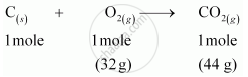CBSE (Science) Class 11CBSE
Share

# Calculate the amount of carbon dioxide that could be produced when 1 mole of carbon is burnt in air. - CBSE (Science) Class 11 - Chemistry

ConceptMole Concept and Molar Masses

#### Question

Calculate the amount of carbon dioxide that could be produced when 1 mole of carbon is burnt in air.

#### Solution

The balanced reaction of combustion of carbon can be written as:-As per the balanced equation, 1 mole of carbon burns in1 mole of dioxygen (air) to produce1 mole of carbon dioxide.

Is there an error in this question or solution?

#### Video TutorialsVIEW ALL 

Solution Calculate the amount of carbon dioxide that could be produced when 1 mole of carbon is burnt in air. Concept: Mole Concept and Molar Masses.
S